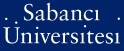# Fast algorithms for smooth and monotone covariance matrix estimation

Corum, Aycan Adrian (2012) Fast algorithms for smooth and monotone covariance matrix estimation. [Thesis]PDF - Registered users only - Requires a PDF viewer such as GSview, Xpdf or Adobe Acrobat Reader1337Kb

## Abstract

In this thesis the problem of interest is, within the setting of financial risk management, covariance matrix estimation from limited number of high dimensional independent identically distributed (i.i.d.) multivariate samples when the random variables of interest have a natural spatial indexing along a low-dimensional manifold, e.g., along a line. Sample covariance matrix estimate is fraught with peril in this context. A variety of approaches to improve the covariance estimates have been developed by exploiting knowledge of structure in the data, which, however, in general impose very strict structure. We instead exploit another formulation which assumes that the covariance matrix is smooth and monotone with respect to the spatial indexing. Originally the formulation is derived from the estimation problem within a convex-optimization framework, and the resulting semidefinite-programming problem (SDP) is solved by an interior-point method (IPM). However, solving SDP via an IPM can become unduly computationally expensive for large covariance matrices. Motivated by this observation, this thesis develops highly efficient first-order solvers for smooth and monotone covariance matrix estimation. We propose two types of solvers for covariance matrix estimation: first based on projected gradients, and then based on recently developed optimal first order methods. Given such numerical algorithms, we present a comprehensive experimental analysis. We first demonstrate the benefits of imposing smoothness and monotonicity constraints in covariance matrix estimation in a number of scenarios, involving limited, missing, and asynchronous data. We then demonstrate the potential computational benefits offered by first order methods through a detailed comparison to solution of the problem via IPMs.

Item Type: Thesis Financial risk management. -- Optimal first-order methods. -- Covariance matrix estimation. -- Semidefinite programming. -- Finansal risk yönetimi. -- Optimal birinci derece yöntemler. -- Ortak değişinti matrisi kestirimi. -- Yarı kesin programlama. T Technology > TK Electrical engineering. Electronics Nuclear engineering > TK7800-8360 Electronics > TK7885-7895 Computer engineering. Computer hardware 24615 IC-Cataloging 29 Sep 2014 15:33 29 Sep 2014 15:33

Repository Staff Only: item control page